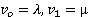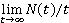### Create an Account

Home / Questions / Suppose that the state of the system can be modeled as a two-state continuous-time Markov ...

# Suppose that the state of the system can be modeled as a two-state continuous-time Markov chain with transition rates When the state of the system is i events occur in accordance

Suppose that the "state" of the system can be modeled as a two-state continuous-time Markov chain with transition ratesWhen the state of the system is i, "events" occur in accordance with a Poisson process with rate a„ i = 0, 1 Let NO) denote the number of events in (0, t)

(a) Find(b) If the initial state is state 0, find E[N(t)].

Aug 05 2020 View more View LessSubscribe To Get Solution